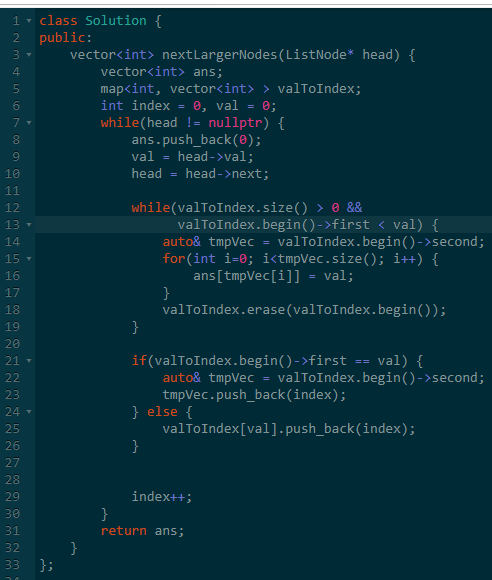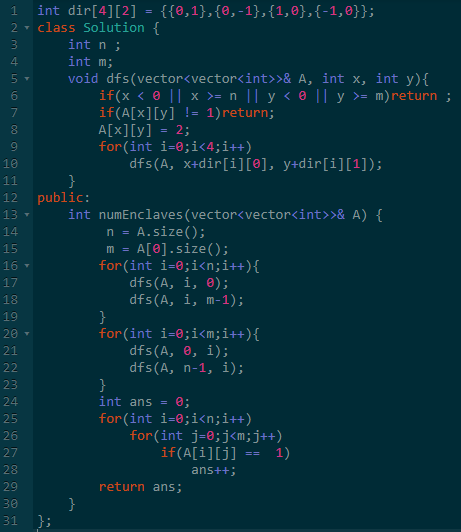# 【算法】Leetcode 第130场比赛回顾

## 一、背景leetcode这次举办比赛的官方人员肯定监听我的QQ算法群的聊天记录了。

## 二、可被 5 整除的二进制前缀

``````pre(i) = pre(i-1)*2 + val[i])
pre(i) % 5
= (pre(i-1)*2 + val[i])%5
= (pre(i-1)% 5)*2 + val[i])%5
``````
``````class Solution {
public:
vector<bool> prefixesDivBy5(vector<int>& A) {
vector<bool> ans;
int now = 0;

for(int i=0; i<A.size(); i++) {
now = (now * 2 + A[i])%5;
if(now ==0) {
ans.push_back(1);
} else {
ans.push_back(0);
}
}
return ans;
}
};
``````

## 三、负二进制转换

`````` (-2)^(2k+2) + (-2)^(2k+1)
=2^(2k+2) - 2^(2k+1)
=2*2^(2k+1) - 2^(2k+1)
=2^(2k+1)
``````

``````string baseNeg2(int N) {
if(N == 0){
return "0";
}
string ans;
int pre = 0;
int bit = 0;

while(N>0 || pre >0){
int sum = N%2 + pre;
if(sum == 0){
ans.push_back('0');
}else if(sum == 1){
////根据奇偶性决定是否进位
pre = bit%2;
ans.push_back('1');
}else if(sum == 2){
pre = 1; //进位
ans.push_back('0');
}
bit++;
N = N/2;
}

std::reverse(ans.begin(), ans.end());
return ans;
}
``````

## 四、链表中的下一个更大节点PS：这里有一个注意事项：位置的值可能重复，所以值相同时，所有的下标都需要保存下来。## 五、飞地的数量-EOF-

## 关注公众号,接收最新消息

1.